How Cheenta works to ensure student success?
Explore the Back-Story

# Letter to parents: Talk about infinityDear parent,

One of the key contributions of modern mathematics is its tryst with infinity. As parents and teachers we can initiate thought provoking communication with our children using infinity.

Consider the following set:

N = {1, 2, 3, … }

Notice that N contains infinitely many elements.

Take a subset of N that consists of multiples of 2. Lets call it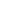.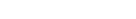Notice again that N1 contains infinitey many elements. Next consider a subset of N1 that contains only the multiples of 3. Lets call that N2.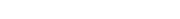Student may say: Isn’t 9 a multiple of 3? Should it not be in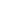?

No. Because we are taking those multiples of 3 which are in. Hence they must be simultaneously multiples of 2 and 3.

Proceeding like this we can create infinitely many sets: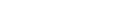These sets are nested! That is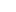containscontainscontainsetc. Moreover each of them contains infinitely many terms.

QUESTION: What is in the intersection of all of these sets? That is : what is common in all of them?

This question provokes the child to really think about infinity. For the finite case it can also make a nice combinatorics problem using method of inclusion and exclusion: how many numbers from 1 to 1000 are multiples of 2 or 3 or both.

In fact this last sentence makes the student worry about the word ‘or’. It is is nice place to introduce exclusive or.

Dr. Ashani Dasgupta

Founder, Cheenta

(Ph.D. in Mathematics from University of Wisconsin, Milwaukee, USA. Research Interest: Geometric Group Theory)

Dear parent,

One of the key contributions of modern mathematics is its tryst with infinity. As parents and teachers we can initiate thought provoking communication with our children using infinity.

Consider the following set:

N = {1, 2, 3, … }

Notice that N contains infinitely many elements.

Take a subset of N that consists of multiples of 2. Lets call it.Notice again that N1 contains infinitey many elements. Next consider a subset of N1 that contains only the multiples of 3. Lets call that N2.Student may say: Isn’t 9 a multiple of 3? Should it not be in?

No. Because we are taking those multiples of 3 which are in. Hence they must be simultaneously multiples of 2 and 3.

Proceeding like this we can create infinitely many sets:These sets are nested! That iscontainscontainscontainsetc. Moreover each of them contains infinitely many terms.

QUESTION: What is in the intersection of all of these sets? That is : what is common in all of them?

This question provokes the child to really think about infinity. For the finite case it can also make a nice combinatorics problem using method of inclusion and exclusion: how many numbers from 1 to 1000 are multiples of 2 or 3 or both.

In fact this last sentence makes the student worry about the word ‘or’. It is is nice place to introduce exclusive or.

Dr. Ashani Dasgupta

Founder, Cheenta

(Ph.D. in Mathematics from University of Wisconsin, Milwaukee, USA. Research Interest: Geometric Group Theory)

This site uses Akismet to reduce spam. Learn how your comment data is processed.

### Knowledge Partner